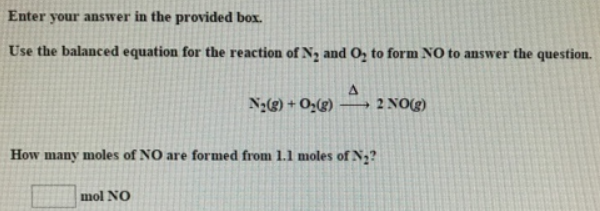# Problem: Use the balanced equation for the reaction of N 2 and O2 to form NO to answer the question. N2 (g) + O2 (g) → 2NO (g) How many moles of NO are formed from 1.1 moles of N 2?

###### FREE Expert Solution
90% (292 ratings)###### Problem Details

Use the balanced equation for the reaction of N 2 and O2 to form NO to answer the question.

N2 (g) + O(g) → 2NO (g)

How many moles of NO are formed from 1.1 moles of N 2